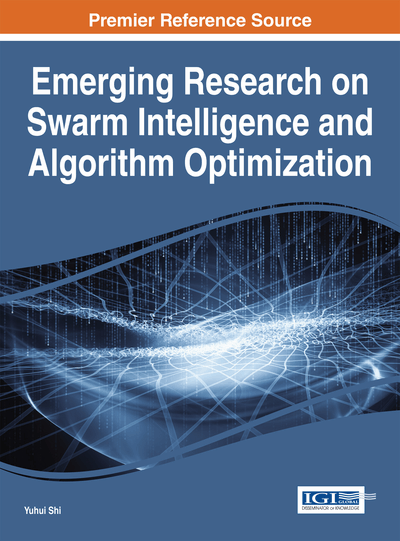# Population Diversity of Particle Swarm Optimizer Solving Single- and Multi-Objective Problems

Shi Cheng (University of Nottingham Ningbo, China), Yuhui Shi (Xi'an Jiaotong-Liverpool University, China) and Quande Qin (Shenzhen University, China)
DOI: 10.4018/978-1-4666-6328-2.ch004

## Abstract

Premature convergence occurs in swarm intelligence algorithms searching for optima. A swarm intelligence algorithm has two kinds of abilities: exploration of new possibilities and exploitation of old certainties. The exploration ability means that an algorithm can explore more search places to increase the possibility that the algorithm can find good enough solutions. In contrast, the exploitation ability means that an algorithm focuses on the refinement of found promising areas. An algorithm should have a balance between exploration and exploitation, that is, the allocation of computational resources should be optimized to ensure that an algorithm can find good enough solutions effectively. The diversity measures the distribution of individuals' information. From the observation of the distribution and diversity change, the degree of exploration and exploitation can be obtained. Another issue in multiobjective is the solution metric. Pareto domination is utilized to compare two solutions; however, solutions are almost Pareto non-dominated for multiobjective problems with more than ten objectives. In this chapter, the authors analyze the population diversity of a particle swarm optimizer for solving both single objective and multiobjective problems. The population diversity of solutions is used to measure the goodness of a set of solutions. This metric may guide the search in problems with numerous objectives. Adaptive optimization algorithms can be designed through controlling the balance between exploration and exploitation.
Chapter Preview
Top

## Introduction

Optimization, in general, is concerned with finding “best available” solution(s) for a given problem within allowable time. In mathematical terms, an optimization problem in, or simply an optimization problem, is a mapping, whereis termed as decision space (Adra, Dodd, & Griffin, et al., 2009), parameter space (Jin & Sendhoff, 2009), or problem space (Weise, Zapf, & Chiong, et al., 2009)), andis termed as objective space (Sundaram, 1996). Optimization problems can be divided into two categories depending on the value of. When, this kind of problems is called Single Objective Optimization (SOO), and when, this is called Multi-Objective Optimization (or Many Objective Optimization, MOO).

Particle Swarm Optimizer/Optimization (PSO), which is one of the evolutionary computation techniques, was invented by Eberhart and Kennedy in 1995 (Eberhart & Kennedy, 1995; Kennedy & Eberhart, 1995). It is a population-based stochastic algorithm modeled on the social behaviors observed in flocking birds. Each particle, which represents a solution, flies through the search space with a velocity that is dynamically adjusted according to its own and its companion's historical behaviors. The particles tend to fly toward better search areas over the course of the search process (Eberhart & Shi, 2001; Hu, Shi, & Eberhart, 2004).

Premature convergence occurs when all individuals in population-based algorithms are trapped in local optima. In this situation, the exploration of algorithm is greatly reduced, i.e., the algorithm is searching in a narrow space. For continuous optimization problems, there are infinite numbers of potential solutions. Even with consideration of computational precision, there still have great number of feasible solutions. The computational resources allocation should be optimized, i.e., maintaining an appropriate balance between exploration and exploitation is a primary factor.

Different problems have different properties. Single objective problem could be divided into unimodal problem and multi-modal problem. As the name indicated, a unimodal problem has only one optimum solution, on the contrary, a multi-modal problem has several or number of optimum solutions, of which many are local optimal solutions. Optimization algorithms are difficult to find the global optimum solutions because generally it is hard to balance between fast converge speed and the ability of “jumping out” of local optimum. In other words, we need to avoid premature in the search process.

Different computational resource allocation methods should be taken when dealing with different problems. A good algorithm should balance its exploration and exploitation ability during its search process. The concept of exploration and exploitation was firstly introduced in organization science (March, 1991; Gupta, Smith, & Shalley, 2006). The exploration ability means an algorithm can explore more search place, to increase the possibility that the algorithm can find “good enough” solution(s). In contrast, the exploitation ability means that an algorithm focuses on the refinement of found promising areas.

## Complete Chapter List

Search this Book:
Reset